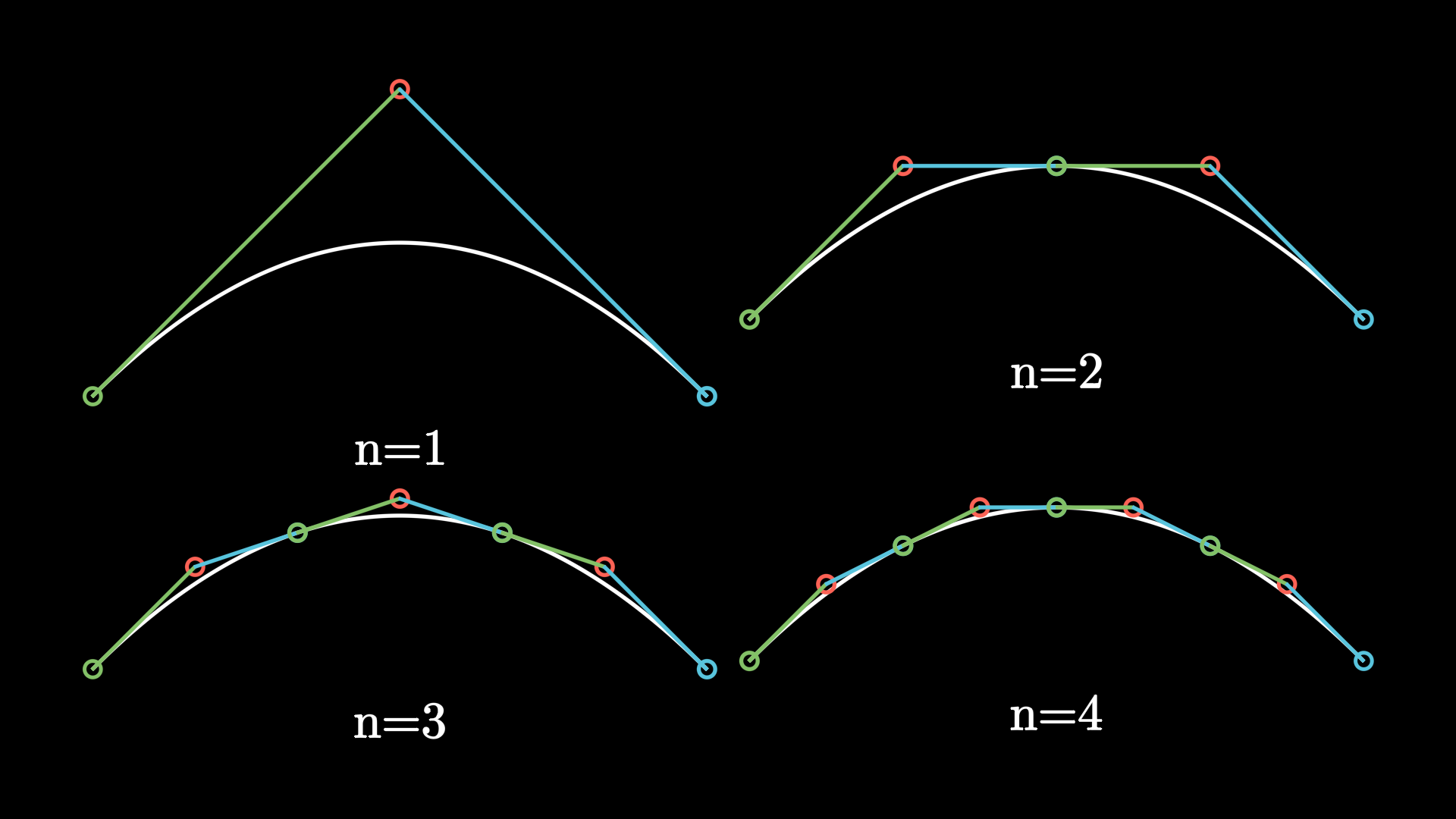# bezier#

Utility functions related to Bézier curves.

Functions

bezier(points)[source]#

Classic implementation of a bezier curve.

Parameters

points (np.ndarray) – points defining the desired bezier curve.

Returns

function describing the bezier curve.

Return type

Callable[[float], Union[int, Iterable]]

diag_to_matrix(l_and_u, diag)[source]#

Converts array whose rows represent diagonal entries of a matrix into the matrix itself. See scipy.linalg.solve_banded

Parameters
• l_and_u (tuple[int, int]) –

• diag (np.ndarray) –

Return type

np.ndarray

get_smooth_cubic_bezier_handle_points(points)[source]#
get_smooth_handle_points(points)[source]#

Given some anchors (points), compute handles so the resulting bezier curve is smooth.

Parameters

points (np.ndarray) – Anchors.

Returns

Computed handles.

Return type

Tuple[np.ndarray, np.ndarray]

integer_interpolate(start, end, alpha)[source]#

Alpha is a float between 0 and 1. This returns an integer between start and end (inclusive) representing appropriate interpolation between them, along with a “residue” representing a new proportion between the returned integer and the next one of the list.

For example, if start=0, end=10, alpha=0.46, This would return (4, 0.6).

Parameters
• start (float) –

• end (float) –

• alpha (float) –

Return type

tuple[int, float]

interpolate(start, end, alpha)[source]#
Parameters
• start (ndarray) –

• end (ndarray) –

• alpha (float) –

Return type

ndarray

inverse_interpolate(start, end, value)[source]#
Parameters
• start (float) –

• end (float) –

• value (float) –

Return type

ndarray

is_closed(points)[source]#
Parameters

points (tuple[np.ndarray, np.ndarray]) –

Return type

bool

match_interpolate(new_start, new_end, old_start, old_end, old_value)[source]#
Parameters
• new_start (float) –

• new_end (float) –

• old_start (float) –

• old_end (float) –

• old_value (float) –

Return type

ndarray

mid(start, end)[source]#
Parameters
• start (float) –

• end (float) –

Return type

float

partial_bezier_points(points, a, b)[source]#

Given an array of points which define bezier curve, and two numbers 0<=a<b<=1, return an array of the same size, which describes the portion of the original bezier curve on the interval [a, b].

This algorithm is pretty nifty, and pretty dense.

Parameters
• points (ndarray) – set of points defining the bezier curve.

• a (float) – lower bound of the desired partial bezier curve.

• b (float) – upper bound of the desired partial bezier curve.

Returns

Set of points defining the partial bezier curve.

Return type

np.ndarray

point_lies_on_bezier(point, control_points, round_to=1e-06)[source]#

Checks if a given point lies on the bezier curves with the given control points.

This is done by solving the bezier polynomial with the point as the constant term; if any real roots exist, the point lies on the bezier curve.

Parameters
• point (Iterable[float | int]) – The Cartesian Coordinates of the point to check.

• control_points (Iterable[Iterable[float | int]]) – The Cartesian Coordinates of the ordered control points of the bezier curve on which the point may or may not lie.

• round_to (float | int | None) – A float whose number of decimal places all values such as coordinates of points will be rounded.

Returns

Whether the point lies on the curve.

Return type

bool

proportions_along_bezier_curve_for_point(point, control_points, round_to=1e-06)[source]#

Obtains the proportion along the bezier curve corresponding to a given point given the bezier curve’s control points.

The bezier polynomial is constructed using the coordinates of the given point as well as the bezier curve’s control points. On solving the polynomial for each dimension, if there are roots common to every dimension, those roots give the proportion along the curve the point is at. If there are no real roots, the point does not lie on the curve.

Parameters
• point (Iterable[float | int]) – The Cartesian Coordinates of the point whose parameter should be obtained.

• control_points (Iterable[Iterable[float | int]]) – The Cartesian Coordinates of the ordered control points of the bezier curve on which the point may or may not lie.

• round_to (float | int | None) – A float whose number of decimal places all values such as coordinates of points will be rounded.

Returns

List containing possible parameters (the proportions along the bezier curve) for the given point on the given bezier curve. This usually only contains one or zero elements, but if the point is, say, at the beginning/end of a closed loop, may return a list with more than 1 value, corresponding to the beginning and end etc. of the loop.

Return type

np.ndarray[float]

Raises

ValueError – When point and the control points have different shapes.

Remaps the number of curves to a higher amount by splitting bezier curves

Parameters
• triplets (Iterable[Iterable[float]]) – The triplets of the quadratic bezier curves to be remapped shape(n, 3, 3)

• new_number_of_curves (int) – The number of curves that the output will contain. This needs to be higher than the current number.

Return type

The new triplets for the quadratic bezier curves.

Split a quadratic Bézier curve at argument t into two quadratic curves.

Parameters
• points (ndarray) – The control points of the bezier curve has shape [a1, h1, b1]

• t (float) – The t-value at which to split the Bézier curve

Returns

• The two Bézier curves as a list of tuples,

• has the shape [a1, h1, b1], [a2, h2, b2]

Return type

ndarray

Subdivide a quadratic Bézier curve into n subcurves which have the same shape.

The points at which the curve is split are located at the arguments $$t = i/n$$ for $$i = 1, ..., n-1$$.

Parameters
• points (Iterable[float]) – The control points of the Bézier curve in form [a1, h1, b1]

• n (int) – The number of curves to subdivide the Bézier curve into

Return type

The new points for the Bézier curve in the form [a1, h1, b1, a2, h2, b2, ...]